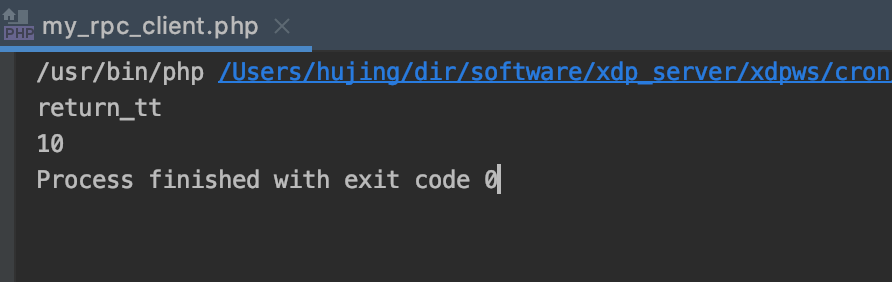# 概述

RPC这个东西是什么? 第一次听说他, 还要在它的前边加个G, 当时我以为GRPC是一项技术, 后来才知道, 并不是这样. GRPC只是RPC的谷歌实现.

# 自己实现

1. 通信问题
2. 定义传输的数据格式
3. 如何封装后可以达到像调用本地函数一样的效果

PHP中进行socket连接十分简单, 直接调用系统函数. 通信问题解决了, 剩下的就是传输数据了, so easy

<?php
class RpcServer{

private $port = 0; // 监听端口号 private$host = ''; // IP

public function __construct($host,$port){
$this->host =$host;
$this->port =$port;
}

/**
* 运行, 监听端口并处理
*/
public function run(){
// 创建socket
$server = stream_socket_server("tcp://{$this->host}:{$this->port}"); if(empty($server)) throw new Exception('创建套接字失败');
// 监听
while (true){
$client = stream_socket_accept($server);
if(empty($client)) continue; // 处理请求$this->disposeClient($client); fclose($client);
}
}

private function disposeClient($client){$buf = fread($client, 4096);$array = json_decode($buf, true); // 创建对象并调用方法$class = $array['class'] ?? '';$method = $array['method'] ?? '';$params = $array['params'] ?? [];$instance = new $class();$result = $instance->$method(...$params); fwrite($client, json_encode($result)); } } // 测试调用类 class Test{ public function tt(){ return 'return_tt'; } public function add($a, $b){ return$a + $b; } } (new RpcServer('127.0.0.1', 8888)) ->run(); 调用方: <?php class RpcClient{ private$urlInfo = null;
private $className = ''; private function __construct($url, $className){$this->urlInfo = parse_url($url);$this->className = $className; } public static function getInstance($className){
return new RpcClient('127.0.0.1:8888', $className); } public function __call($name, $arguments){ // 创建客户端$client = stream_socket_client("tcp://{$this->urlInfo['host']}:{$this->urlInfo['port']}");
if(empty($client)) return null; // 发送数据 fwrite($client, json_encode([
'class' => $this->className, 'method' =>$name,
'params' => $arguments, ])); // 接收返回$data = fread($client, 4096); // 关闭客户端 fclose($client);
return json_decode($data, true); } }$test = RpcClient::getInstance('Test');
echo $test->tt(), PHP_EOL; echo$test->add(4, 6);# 继续

nice, 自己做完了, 对RPC是个什么东西有了一个基本的概念.

WHAT

RPC是什么? 简单说, 就是远程函数调用. 字面意思, 很好理解.

WHY

HOW

1. 网络通信
2. 信息格式
3. 对象状态保存

1.网络通信

2.信息格式

3.对象状态保存

1. 客户端创建RPC对象
2. 客户端调用方法
3. RPC解析方法并将对象及参数做序列化
4. RPC通过网络连接发送方法调用
5. 服务端接收到方法调用, 解析对象及参数反序列化
6. 服务端执行方法并将结果序列化返回
7. 客户端接收到结果并进行解析, 返回给本地调用者
8. 拿到最终结果

RPC适用于内部网络不同项目之间的通信, 如果是对外暴露的, 个人感觉还是通过接口的形式吧.0 评论

0